1. 清华大学 汽车工程系, 汽车安全与节能国家重点实验室, 北京 100084 ;
2. 北京机电工程总体设计部, 北京 100854

Collaborative optimization of discrete structures based on a substructuring method
ZHONG Wei1 , SU Ruiyi2 , GUI Liangjin1 , FAN Zijie11. State Key Laboratory of Automotive Safety and Energy, Department of Automotive Engineering, Tsinghua University, Beijing 100084, China ;
Abstract:A decomposition strategy based on substructuring is developed for optimizing complex discrete structures with a large number of design variables and a collaborative architecture is used as the solver. A large structure is decomposed into several small substructures with no overlap, where each substructure corresponds to a discipline. The variables, objectives and constraints in the original problem are assigned to the separate disciplines. Only one finite element analysis of the complete structure is performed at the system level during each iteration with optimization and finite element analyses of the substructures at the discipline levels. Coupled state variables are passed from the system level to the disciplines as constants. The coupled state variables are updated after each finite element analysis of the complete structure at the system level to coordinate the differences in the coupled state variables among disciplines. Thus, a complex structural optimization problem is decomposed into several parallel, self-governed subproblems. The results of numerical examples demonstrate that this cooperative optimization method requires less evaluations of the complete structure and is able to obtain better results with better stability than optimizing the complete structure as a whole.
Key words: discrete structure     structural optimization     substructuring method     collaborative optimization

1 标准协同优化算法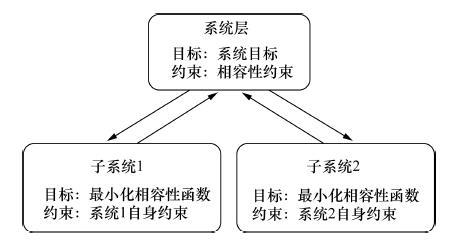图 1 标准协同优化算法的基本框图

 \begin{align} & \min f(X,Y) \\ & s.t.g\left( X,Y \right)\le 0, \\ & Y=h\left( X \right), \\ & {{X}_{\min }}\le X\le {{X}_{\max }}. \\ \end{align} (1)

 \begin{align} & \min {{J}_{i}}=\left\| {{X}_{\text{sh}i}}-{{Z}_{\text{sh}i}} \right\|_{2}^{2}+\left\| {{Y}_{ji}}-{{Z}_{ji}} \right\|_{2}^{2}+ \\ & \left\| {{Y}_{ik}}-{{Z}_{ik}} \right\|_{2}^{2} \\ & \text{s}\text{.t}\text{.}{{g}_{i}}\left( {{X}_{i}} \right)\le 0, \\ & {{Y}_{ik}}={{h}_{i}}\left( {{X}_{i}} \right), \\ & {{X}_{i,\min }}\le {{X}_{i}}\le {{X}_{i,\max }}. \\ \end{align} (2)

 \begin{align} & \min f\left( Z \right) \\ & \text{s}\text{.t}\text{.}J_{i}^{*}\le \varepsilon ,i=1,2,\cdots ,{{N}_{\text{d}}}, \\ & {{Z}_{\min }}\le Z\le {{Z}_{\max }}. \\ \end{align} (3)

2 离散结构优化问题描述

 \begin{align} & \min W \\ & \text{s}\text{.t}\text{.}\left| {{\sigma }_{i}} \right|\le {{\sigma }_{u}},i=1,2,\cdots ,{{N}_{\text{L}}}, \\ & {{x}_{\min }}\le {{x}_{j}}\le {{x}_{\max }},j=1,2,\cdots ,N. \\ \end{align} (4)

 $KU=F.$ (5)

3 基于子结构分解的协同优化框架 3.1 基于子结构方法的分解策略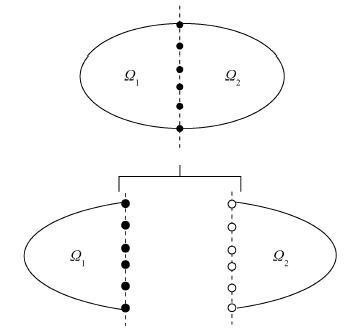图 2 结构分解示意图

I表示子结构的内部节点集合,B表示子结构的外部节点集合,则整体刚度矩阵和第i个子结构的刚度矩阵分别为：

 $K=\left[ \begin{matrix} K_{II}^{\left( 1 \right)} & K_{IB}^{\left( 1 \right)} & O \\ K_{BI}^{\left( 1 \right)} & K_{BB}^{\left( 1 \right)}+K_{BB}^{\left( 2 \right)} & K_{IB}^{\left( 2 \right)} \\ O & K_{BI}^{\left( 2 \right)} & K_{II}^{\left( 2 \right)} \\ \end{matrix} \right],$ (6)
 ${{K}^{\left( i \right)}}=\left[ \begin{matrix} K_{II}^{\left( i \right)} & K_{IB}^{\left( i \right)} \\ K_{BI}^{\left( i \right)} & K_{BB}^{\left( i \right)} \\ \end{matrix} \right].$ (7)

 $\left[ {\matrix{ {K_{II}^{\left( i \right)}} & {K_{IB}^{\left( i \right)}} \cr {K_{BI}^{\left( i \right)}} & {K_{BB}^{\left( i \right)}} \cr } } \right]\left[ \matrix{ U_I^{\left( i \right)} \hfill \cr U_B^{\left( i \right)} \hfill \cr} \right] = \left[ \matrix{ F_I^{\left( i \right)} \hfill \cr F_B^{\left( i \right)} \hfill \cr} \right].$ (8)

 ${{\tilde{K}}^{\left( i \right)}}U_{B}^{\left( i \right)}={{\tilde{F}}^{\left( i \right)}}.$ (9)

 ${{\tilde{K}}^{\left( i \right)}}=K_{BB}^{\left( i \right)}-K_{BI}^{\left( i \right)}{{\left[ K_{II}^{\left( i \right)} \right]}^{-1}}K_{IB}^{\left( i \right)},$ (10)
 ${{\tilde{F}}^{\left( i \right)}}=F_{B}^{\left( i \right)}-K_{BI}^{\left( i \right)}{{\left[ K_{II}^{\left( i \right)} \right]}^{-1}}F_{I}^{\left( i \right)}.$ (11)

3.2 系统层和学科层构造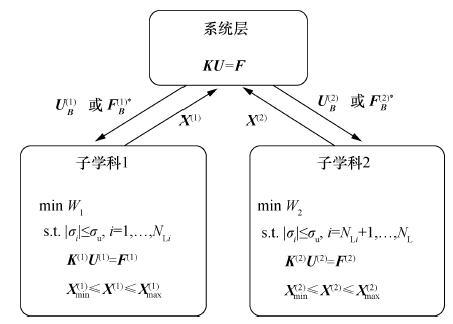图 3 本文的离散结构协同优化算法框图(两个子学科)

3.3 算法流程

1) 设k=0,给定初值X0;

2) 系统层进行整体结构分析,求得结构内部边界条件UB*FB*;

3) 将UB*/FB*作为定值传递至学科i,从Xk中提取Xk(i)作为学科i的初值;

4) 优化学科i,获得第k次系统迭代时学科i的最优解Xk(i)opt;

5) 是否达到最大迭代次数？是则转7),否则转6);

6) k=k+1,Xk=[Xk-1(1)opt Xk-1(2)opt],将Xk作为定值传入系统层,转2);

7) 结束。

4 算 例

4.1 20-杆桁架结构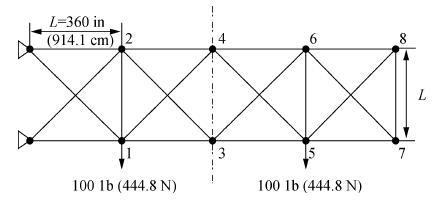图 4 20-杆桁架结构

 设计变量 初值 最优解 1 2 3 4 1 2 3 4 x1/in2 14 15 10 5 15 15 15 15 x2/in2 14 15 10 5 9.001 8.999 9.001 9.000 x3/in2 9 15 10 5 0.1 0.1 0.1 0.1 x4/in2 9 15 10 5 9.898 9.899 9.898 9.898 x5/in2 9 15 10 5 1.788 1.786 1.789 1.788 x6/in2 9 15 10 5 4.958 4.957 4.959 4.958 x7/in2 9 15 10 5 7.042 7.042 7.042 7.042 x8/in2 9 15 10 5 4.301 4.302 4.301 4.301 x9/in2 9 15 10 5 1.653 1.651 1.654 1.653 x10/in2 9 15 10 5 1.110 1.110 1.110 1.110 x11/in2 9 15 10 5 3.906 3.906 3.906 3.906 x12/in2 9 15 10 5 0.1 0.1 0.1 0.1 x13/in2 9 15 10 5 5.523 5.523 5.523 5.523 x14/in2 9 15 10 5 0.133 0.133 0.133 0.133 x15/in2 9 15 10 5 0.1 0.1 0.1 0.1 x16/in2 9 15 10 5 0.1 0.1 0.1 0.1 x17/in2 9 15 10 5 0.1 0.1 0.1 0.1 x18/in2 9 15 10 5 0.1 0.1 0.1 0.1 x19/in2 9 15 10 5 0.1 0.1 0.1 0.1 x20/in2 9 15 10 5 0.1 0.1 0.1 0.1 是否可行 是 是 否 否 是 是 是 是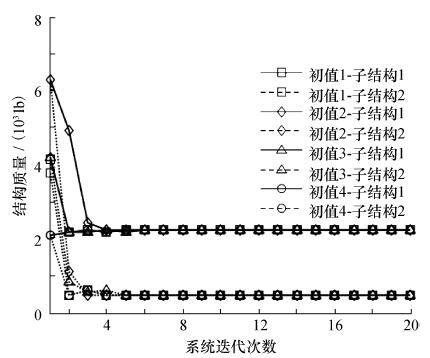图 5 20-杆桁架结构子结构质量迭代曲线

 最优解总质量/lb 整体结构分析次数 学科1结构分析次数 学科2结构分析次数 总时间/s 协同优化 初值1 2 694.4 8 818 489 398.1 初值2 2 694.2 6 674 471 321.6 初值3 2 694.5 10 967 848 458.8 初值4 2 694.4 8 731 453 332.5 整体优化 初值1 2 697.3 964 460.2 初值2 2 697.2 625 305.5 初值3 2 741.6 489 249.2 初值4 2 712.7 674 319.4

4.2 72-杆空间桁架结构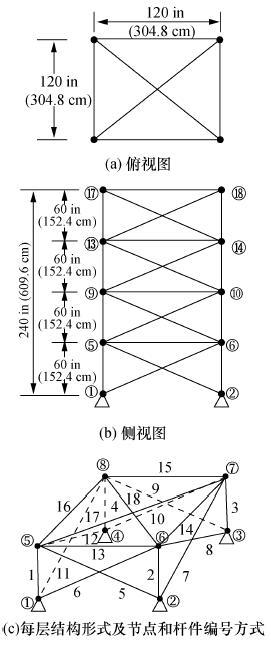图 6 72-杆空间桁架结构

 节点 工况1 工况2 Px/kips Py/kips Pz/kips Px/kips Py/kips Pz/kips 17 5.0 5.0 -5.0 0.0 0.0 -5.0 18 0.0 0.0 0.0 0.0 0.0 -5.0 19 0.0 0.0 0.0 0.0 0.0 -5.0 20 0.0 0.0 0.0 0.0 0.0 -5.0

 子结构 结构内部边界条件 1 F5—F8 2 U5—U8,F9—F12 3 U9—U12,F13—F16 4 U13—U16

 设计变量 初值 最优解 1 2 1 2 x1/in2 0.1 3.0 0.293 0.295 x2/in2 0.1 3.0 0.1 0.1 x3/in2 0.1 3.0 0.1 0.1 x4/in2 0.1 3.0 0.1 0.1 x5/in2 0.1 3.0 0.197 0.199 x6/in2 0.1 3.0 0.1 0.1 x7/in2 0.1 3.0 0.1 0.1 x8/in2 0.1 3.0 0.1 0.1 x9/in2 0.1 3.0 0.191 0.191 x10/in2 0.1 3.0 0.1 0.1 x11/in2 0.1 3.0 0.1 0.1 x12/in2 0.1 3.0 0.1 0.1 x13/in2 0.1 3.0 0.187 0.189 x14/in2 0.1 3.0 0.127 0.1 x15/in2 0.1 3.0 0.1 0.1 x16/in2 0.1 3.0 0.1 0.1 是否可行 否 是 是 是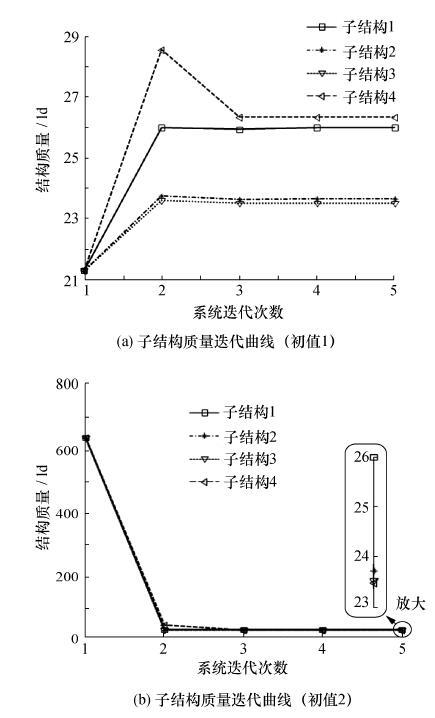图 7 72-杆空间桁架结构子结构质量迭代曲线

 对比项 最优解总质量/lb 整体结构分析次数 学科1结构分析次数 学科2结构分析次数 学科3结构分析次数 学科4结构分析次数 总时间/s 协同优化 初值1 99.439 3 70 96 78 122 58.95 初值2 96.685 3 131 117 78 180 83.3 整体优化 初值1 680.26 173 — 初值2 104.13 157 78.4

5 结论

  Kroo I, Altus S, Braun R, et al. Multidisciplinary optimization methods for aircraft preliminary design [C] // Proc of 5th AIAA/USAF/NASA/ISSMO Symposium on Multidisciplinary Analysis. Panama City Beach, FL: AIAA Press, 1994: 697-707.  LI Haiyan, MA Mingxu, ZHANG Wenlei. Improving collaborative optimization for MDO problems with multi-objective subsystems[J]. Structural and Multidisciplinary Optimization,2014, 49 (4) : 609 –620.  王平, 郑松林, 吴光强. 基于协同优化和多目标遗传算法的车身结构多学科优化设计[J]. 机械工程学报,2011, 47 (2) : 102 –108. WANG Ping, ZHENG Songlin, WU Guangqiang. Multidisciplinary design optimization of vehicle body structure based on collaborative optimization and multi-objective genetic algorithm[J]. Journal of Mechanical Engineering,2011, 47 (2) : 102 –108. (in Chinese)  李响, 李为吉, 柳长安. 一种基于几何分析的协同优化方法[J]. 机械工程学报,2010, 46 (7) : 142 –147. LI Xiang, LI Weiji, LIU Changan. Novel collaborative optimization method based on geometric analysis[J]. Journal of Mechanical Engineering,2010, 46 (7) : 142 –147. (in Chinese)  苏瑞意, 桂良进, 吴章斌, 等. 大客车车身骨架多学科协同优化设计[J]. 机械工程学报,2010, 46 (18) : 128 –133. SU Ruiyi, GUI Liangjin, WU Zhangbin, et al. Multidisciplinary design and collaborative optimization for bus body[J]. Journal of Mechanical Engineering,2010, 46 (18) : 128 –133. (in Chinese)  Balling R J, Sobieszczanski-Sobieski J. An algorithm for solving the system-level problem in multilevel optimization[J]. Structural Optimization,1995, 9 (3) : 168 –177.  Lan F, Chen J, Lin J. Comparative analysis for bus side structures and lightweight optimization[J]. Proceedings of the Institution of Mechanical Engineers Part D: Journal of Automobile Engineering,2004, 218 (10) : 1067 –1075.  彭磊, 刘莉, 龙腾. 基于径向基函数的卫星平台桁架结构优化设计[J]. 南京航空航天大学学报,2014, 46 (3) : 475 –480. PENG Lei, LIU Li, LONG Teng. Satellite bus truss structure optimization using radial basis function[J]. Journal of Nanjing University of Aeronautics & Astronautics,2014, 46 (3) : 475 –480. (in Chinese)  唐和生, 范德伟, 王兆亮, 等. 桁架尺寸优化微分演化算法[J]. 湖南大学学报: 自然科学版,2011, 38 (11) : 13 –18. TANG Hesheng, FAN Dewei, WANG Zhaoliang, et al. Differential evolution algorithm to size the optimization of truss structures[J]. Journal of Hunan University: Natural Sciences,2011, 38 (11) : 13 –18. (in Chinese)  Azad S K, Ebi O H. Discrete sizing optimization of steel trusses under multiple displacement constraints and load cases using guided stochastic search technique[J]. Structural and Multidisciplinary Optimization,2015, 52 (2) : 383 –404.  SU Ruiyi, GUI Liangjin, FAN Zijie. Truss topology optimization using genetic algorithm with individual identification [C] // Proc of the World Congress on Engineering. London, UK, 2009: 1089-1093.  马少坤, 于淼, 崔皓东. 子结构分析的基本原理和ANSYS软件的子结构分析方法[J]. 广西大学学报: 自然科学版,2004, 29 (2) : 150 –153. MA Shaokun, YU Miao, CUI Haodong. The basic principle of substructure analysis and the technique of substructure analysis with the software of ANSYS[J]. Journal of Guangxi University: Nat Sci Ed,2004, 29 (2) : 150 –153. (in Chinese)  Amir H G, YANG Xinshe. Benchmark problems in structural optimization [C]// Slawomir K, YANG Xinshe. Computational Optimization, Methods and Algorithms. Berlin: Springer-Verlag, 2011: 259-279.  Nair P B, Keane A J. Coevolutionary architecture for distributed optimization of complex coupled systems[J]. AIAA Journal,2002, 40 (7) : 1434 –1443.  Sedaghati R. Benchmark case studies in structural design optimization using the force method[J]. International Journal of Solids and Structures,2005, 42 (21/22) : 5848 –5871.